# No voltage gain in a common emitter class A amplifier ngspice Simulation

#### Man789

Joined Jan 26, 2023
10
No voltage gain in a common emitter class A amplifier using a 3904 NPN transistor - ngspice Simulation

Hi everybody!

I am currently trying to build an amplifier for a condenser microphone in order to use it as a way to amplify an acoustic guitar, nonetheless, as expected, I am having some troubles to really achieve my goals.

In order to learn I decided to make some analogue simulations using QUCS-Spice and the ngspice simulator.

I copied a Common Emitter Class A amplifier from internet and I tried to bias the transistor 2N3904 in order to achieve an amplification in current as well as in voltage in the output, which will be a 35 Ohms old ear-speaker.

Nonetheless there is something I cannot achieve independently of the resistors and capacitors used for the common emitter class a amplifier circuit, and would like to ask how I can improve the voltage gain of the circuit, in order to amplify the power gain within it.

In order to explain myself i will post some pictures with two different resistors and their output, following ngspice simulation results.

In order to amplify the signal from a condenser microphone, model KPCM-G97H45P-43DB-1187, using a battery of 9 Volts as power supply I decided to use a resistor for supplying the power to the microphone condenser of 14.7k in series with the microphone and a 220 uF capacitor in order to decouple the DC signal and send it to the amplifier circuit, correct me if this is not the right verb (decouple).

Using just the microphone within the 9 Volt power source it is possible to see the signal in an audio recording software as Audacity, but the signal is quite low, I can just see like a flat line without any wave looks.

- Circuit Number 1 - "Normal" Resistor selection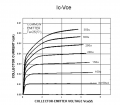For the first biasing point I used the characteristics plot above from a data-sheet found online.
I choose 0.04 A as closed switch current for the transistor, V_CE=0 and made R_C=3/4*R_Total
and R_E = 1/4*R_Total. Therefore:
R_Total = V_CC / I = 9 / 0.04 = 225 Ohms
R_C = 3/4 * R_Total = 168.75
R_E = 1/4 *R_Total = 56.25
From here I selected a Q-Point of I_Base = 100 uA with approximately a collector current of 0.02 A, following the graph above.

Using I_Collector = 0.02 A and a Beta of 300, because of the transistor configuration in ngspice:
I_Base = I_Collector / Beta = 0.02/300 = 6.67 x10^⁻5 A
Using a rule of thumb: I_R1 = 10*I_Base:
I_R1 = 10 * 6.67 x10⁻5 A = 6.67 x10^⁻4 A or I_R1 = 0.667 mA
Using this current and knowing V_BE approx 0.6:
V_R2 = V_BE + R_E*I_E
V_R2 = V_BE + R_E*(I_C+I_B)
I_C approx I_C + I_B
Therefore
V_R2 = V_BE + R_E * I_C
R_2 * I_R2 = V_BE + R_E * I_C
or
R_2 * (I_R1 - I_B) = V_BE + R_E * I_C
Since I_R1 - I_B approx I_R1:
R_2 * I_R1 = V_BE + R_E * I_C
R_2 = (V_BE + R_E * I_C) / I_R1
R_2 = (0.6 + 56.25*0.02) / (6.67 x10^⁻4) = 2586.20 ohms
R1 = V_R1 / I_R1 = (9 - 2586.20 * 6.67 x10^⁻4) / (6.67 x10^⁻4) = 10907.053 Ohms

Here the circuit: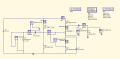And here the simulated output: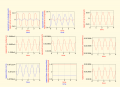My question is why the voltage is not amplified by the circuit and just the current is.

I made some calculus in a excel and it gave my a power gain of around 3,3. I was wondering if it is possible to make it higher or this type of circuit is not design for that use. If so, which type of amplifier do you recommend me to use?

- Circuit Number 2 - Try for Max Current Gain Resistor selection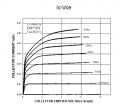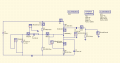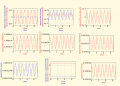For this circuit I tried to select the resistors in order to set the Q-Point as high as possible
I tried to take into account that while the current base increases the same difference in base current relates to a higher difference in collector current.

Ergo:
As I_B increase -> delta I_C / delta I_B increases

So I used a I_C when V_EC = 0 of 0.06 A and a I_C for Q-point of 0.04 A.

Nonetheless the voltage gain seem the same..

Furthermore I build both circuits with the closest real resistors possible and even a second stage, with the same resistor values as the first, and it does not seem a useable amplifier for the microphone, the signal increases but not as much as I expected in the beginning of this journey.

1. Which circuit design I should used in order to amplify the voltage signal as well as the current signal?
2. Which type of amplifiers do you recommend for low noise output?
3. How it is possible to avoid the feedback effect of a loudspeaker next to a microphone?
(I used the circuit using a guitar amp and at 1/3 max gain, where the microphone was next to it, it started piping with no end of the peeeep until it was switched off)
4. It is normal a 3 power gain for a beta of 300?
5. Something wrong in my calculus? Just started with it so there will be a ton of misunderstandings by my part, glad to be corrected.
6. I used a V_BE of 0,6 volts because the transistor is made of s ilicon. Nonetheless in ngspice this value cannot be found anywhere. The nearest value I was able to find for this model was the base-emitter junction built in potential, Vje = 0,75 V or the substrate junction built in potential, Vjs = 0,75 V.
Which one of this values would refer to the V_BE of the transistor? Or it is in ngspice with other parameters calculated?
7. How do I choose correctly the bypass capacitors between signal and amplifier and ampflifier and Load? Any formula over there I was unable to find?

Thanks for your reading and have a nice day. All help or recommendations will be welcome.

#### Attachments

Last edited by a moderator:

#### Ian0

Joined Aug 7, 2020
7,041
1) That circuit will work, with the right choice of resistors.
2) It depends on your source impedance. JFET for a high source impedance, Bipolar for a low source impedance. Never MOSFET
3) By moving the microphone, or using a cardioid-response microphone, correctly aligned with the speaker.
4) That circuit has a gain of 3, it is R4/R5.
7) C>1/(2πfR)

•Man789

#### MrChips

Joined Oct 2, 2009
28,155

•sghioto and Man789

#### Ian0

Joined Aug 7, 2020
7,041
The output impedance is approximately equal to R2.
You will dramatically increase the gain if you put a capacitor across R5. The gain is approximately equal to the impedance at the collector divided by the impedance at the emitter. But in the process you will increase the distortion.

What's your objective here? Are you intending fully to understand the common emitter amplifier? Or is it your objective to make an amplifier for a condensor microphone?

#### Man789

Joined Jan 26, 2023
10
Thanks! I will try again with the output resistance thumb rule and post the results.

The problem with JFET is that there are no power JFET, or they are quite difficult to find, I will try with Bipolar and maybe with MOSFET in order to see the differences between them.

I did already move the microphone to make the test, I was wondering if there was a way to delete the feedback, as the output sound is a quite high frequency sound. Maybe I try with a band pass filter just for fun. Do you think it could work?

- Low Band Pass Filter Question:

About the band pass filter I will try to apply the following circuit I found online, the passive low pass filter: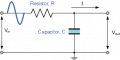Since I am not working with operational amplifiers yet, I will try with the passive low band pass filter for a cut frequency of 8 kHz and 5 kHz in order to try to avoid the high frequency sound band.

I found this formulas online:

X_C = 1 / (2*Pi*f*C)
V_Out = V_In * X_C / ( sqrt( R^2 + X_C ^2) )

and X_C = R is the point where for the higher possible frequency also called cut-off or corner frequency.

On this point the output voltage is attenuated with a gain of -3db or 0.707 voltage gain

Using the formula

Gain in dB = 20 log (V_Out/V_In)
-3 dB = 20 log (V_out / V_In)
V_Out = 10^(-3 dB / 20) * V_In

For a V_In of 1 mV:
V_Out = 10^(-3 dB / 20) * 0.001
V_Out = 7.07945 x10^-4 V = 0,707 mV

So the cut off frequency is the frequency on which the output gain is reduced by 0.707 or -3db.

I have a question about the low pass filter, and it is how to select the value of the first resistance.

Selecting a value of 4.7 kOhms for the first resistance:

X_C = R (at cut off frequency) in order to achieve -3dB gain:
Using: X_C = 1 / (2*Pi*f*C)

- For 8 k Hz and R = 4.7 Ohms

C = 1 / (2*Pi*f*X_C) = 1 / (2*Pi*8000*4.7 kOhms )
C = 1.4109 x 10^-6 F = 1.4109 uF

- For 5 k Hz and R = 4.7 Ohms

C = 1 / (2*Pi*f*X_C) = 1 / (2*Pi*5000*4.7 kOhms )
C = 2.257 x 10^-6 F = 2.257 uF

Nonetheless I wonder how to select the value for the resistance. I guess it has something to do with the input or output impedance of the device, or the relationship between the output impedance and the load. It is also the same as for the amplifier? That the output impedance of the filter needs to be at much as 1/10 of the load input resistance?

Being the output impedance the resistance of the resistor?

What I have found about the resistance is that in 2nd order low pass filters the impedance of the second stage, and each following stage, should be 10 times the impedance of the previous stage. Then:

R_2 = 10 * R_1
C_2 = 1/10 * C_1

My question would be how to choose the impedance for the first stage? I guess it depends on the load connected to the circuit and the load needs to have a input impedance of 10 times the output impedance of the bypass filter. Or it is the opposite? Having the load a 1/10 input impedance compared with the output impedance of the filter?

My goal with the post is to understand how transistors work and why I did not get voltage gain at the output. I just realized that the circuit works as a regulated current source instead of a regulated voltage source. Furthermore I want to build some electronic devices in order to learn about this wonderful science and try to spend less than what I would by buying the components already designed and built.

Now I am focused on understanding amplifiers as well as power supplies or voltage regulators. My goal is to make a good microphone circuit in order to amplify any acoustic or classical guitar and build some loudspeakers amplifiers in order to listen to the differences between class A, class AB and the several types of transistors.

Furthermore the post is not just in order to ask doubts, but also in order to explain what I think I already know. It helps me to understand better the topic and to discover possible errors.

Another question would be if you have any way to calculate the distortion of an amplifier? Also a book name or a link to a web with a good explanation would be great to have.

About cardioid-response microphone, I read some info about them online, thanks for pointing the name by the way. As I understood what is different is the mechanical design, which avoid sound from other directions that are not the frontal. Does this mean that I can build a cardioid-response microphone using a condenser mic? And I just need to build somehow the object/support where it is build in? If so... do you know any good source about design of this type of microphones? Would be really nice to have.

#### Man789

Joined Jan 26, 2023
10
"The output impedance is approximately equal to R2."
With R2 do you mean the resistance connected to the Emitter of the transistor? Or the resistance named R2 in the image of the simulated circuit, otherwise said the second resistance of the voltage divider?

#### Ian0

Joined Aug 7, 2020
7,041
"The output impedance is approximately equal to R2."
With R2 do you mean the resistance connected to the Emitter of the transistor? Or the resistance named R2 in the image of the simulated circuit, otherwise said the second resistance of the voltage divider?
Yiu have different R2s in different drawings. I mean the collector resistor.

Why do you need a power transistor? This is a signal amplifier.
If you think you might be going to drive a loudspeaker, or even headphones, think again.

#### Ian0

Joined Aug 7, 2020
7,041
I did already move the microphone to make the test, I was wondering if there was a way to delete the feedback, as the output sound is a quite high frequency sound. Maybe I try with a band pass filter just for fun. Do you think it could work?
Unfortunately not, there will always be a frequency at which the phase shift is 180° which will vary with the distance between mic and speaker.
If the gain around the loop is greater than 1 it will oscillate.
”Feedback destroyers” exist which look for the peak in the response using FFTs, then introduce a sharp notch filter at that frequency.
Another question would be if you have any way to calculate the distortion of an amplifier? Also a book name or a link to a web with a good explanation would be great to have.
It can calculate distortion. I don‘t know how accurate it is in real life, but it would let you compare two different designs.

About cardioid-response microphone, I read some info about them online, thanks for pointing the name by the way. As I understood what is different is the mechanical design, which avoid sound from other directions that are not the frontal. Does this mean that I can build a cardioid-response microphone using a condenser mic? And I just need to build somehow the object/support where it is build in? If so... do you know any good source about design of this type of microphones? Would be really nice to have.
You would have to find a condenser mic insert with a cardioid response. They are rare but I think I have seen one Once. As condenser mic inserts were designed for telephone handsets, there is very little need for a cardioid response.
Microphones for music use have a much higher spec, especially on noise.

•Man789

#### Man789

Joined Jan 26, 2023
10
Yiu have different R2s in different drawings. I mean the collector resistor.

Why do you need a power transistor? This is a signal amplifier.
If you think you might be going to drive a loudspeaker, or even headphones, think again.
Okay, as I understand now in order to calculate the Output impedance of the amplifier it is needed to short cut the voltage source and open the transistor. So there is no current through the transistor and the impedance it is considered just the R_Collector because the difference of resistance between the short-cut voltage source (approx 0 Ohms) and the resistor voltage divider is really high and it is considered no current through the R_1 and R_2.
In case there was a R_Source then the output impedance would be:
R_Out = R_C + 1 / ((1/R_1 + R_2)+1/R_Source)
Would it be correct?

I do not plan to use the same resistor values, neither the same transistors or amplifier types for the loudspeakers amplifier. I just prefer to do the microphone amplifier first in order to learn all I can about electronics and avoid burning my electrical installation. I need to check cable dimensions in order to know which maximal power can I use and so on.

#### Ian0

Joined Aug 7, 2020
7,041
Okay, as I understand now in order to calculate the Output impedance of the amplifier it is needed to short cut the voltage source and open the transistor. So there is no current through the transistor and the impedance it is considered just the R_Collector because the difference of resistance between the short-cut voltage source (approx 0 Ohms) and the resistor voltage divider is really high and it is considered no current through the R_1 and R_2.
In case there was a R_Source then the output impedance would be:
R_Out = R_C + 1 / ((1/R_1 + R_2)+1/R_Source)
Would it be correct?
Which diagram do your resistor numbers refer to?

#### Man789

Joined Jan 26, 2023
10
Unfortunately not, there will always be a frequency at which the phase shift is 180° which will vary with the distance between mic and speaker.
If the gain around the loop is greater than 1 it will oscillate.
”Feedback destroyers” exist which look for the peak in the response using FFTs, then introduce a sharp notch filter at that frequency.
Great, as I understand it the feedback goes higher as the phase shift is closer to 180º. There is a way to modify the phase shift on purpose? I mean if I could shift the phase shift of the signal from one cycle to another wouldn't it reduce the distortion effect?

It can calculate distortion. I don‘t know how accurate it is in real life, but it would let you compare two different designs.
I would like to stick with ngspice. I just like to use open source software which I can modify if needed. Furthermore there is also distortion simulation and noise simulation for ngspice. (Did not know before) I do not know how it is in comparison with LTSpice but it will make the job for the moment.
If you are interested I have found some official documentation about it:

Section 5.2 - Distortion simulation
Section 5.3 - Noise Simulation

You would have to find a condenser mic insert with a cardioid response. They are rare but I think I have seen one Once. As condenser mic inserts were designed for telephone handsets, there is very little need for a cardioid response.
Microphones for music use have a much higher spec, especially on noise.
Great! I will start searching more about microphones. My knowledge about the topic is at best limited. Thanks for the vocabulary introduction. It always helps a lot.

#### Man789

Joined Jan 26, 2023
10
Which diagram do your resistor numbers refer to?
Sorry... none of them, just the "standard" reference:
R_1 -> Resistor Biasing top (before Base)
R_2 -> Resistor Biasing bottom (after Base)
R_C -> Resistor Collector
R_E -> Resistor Emitter
R_Souce -> Resistor in series with Source ( Not shown in any circuit) (Just for fun calculation)

For future post I will try to use the same references in all the circuits, I know is highly confusing.

#### Ian0

Joined Aug 7, 2020
7,041
Sorry... none of them, just the "standard" reference:
R_1 -> Resistor Biasing top (before Base)
R_2 -> Resistor Biasing bottom (after Base)
R_C -> Resistor Collector
R_E -> Resistor Emitter
R_Souce -> Resistor in series with Source ( Not shown in any circuit) (Just for fun calculation)

For future post I will try to use the same references in all the circuits, I know is highly confusing.
Ignoring the input signal.
You have a fixed voltage at the transistor base, it is Vsupply*R_2/(R_1+R_2).
That voltage less Vbe appears across R_E, so the transistor behaves as a constant current source.
The output impedance of a constant current source is ∞.
The constant current source is in parallel with R_C,
so the output impedance is R_C in parallel with ∞, which equals R_C.

•Man789

#### Man789

Joined Jan 26, 2023
10
Ignoring the input signal.
You have a fixed voltage at the transistor base, it is Vsupply*R_2/(R_1+R_2).
That voltage less Vbe appears across R_E, so the transistor behaves as a constant current source.
The output impedance of a constant current source is ∞.
The constant current source is in parallel with R_C,
so the output impedance is R_C in parallel with ∞, which equals R_C.
Okay, so, if I am not wrong, in an hypothetical circuit as below:The Z_Out would be as mentioned:
Z_Out = R_Collector + 1 / [ 1 / (R_1 + R_2) +1/R_Source ]

*Resistor values are random.

Making a shortcut for the V_CC and open circuit for the transistor, as it works as a current source.

PD: I will make some experiments and try some circuits and come back tomorrow with more questions.

#### Ian0

Joined Aug 7, 2020
7,041
Okay, so, if I am not wrong, in an hypothetical circuit as below:
View attachment 286200

The Z_Out would be as mentioned:
Z_Out = R_Collector + 1 / [ 1 / (R_1 + R_2) +1/R_Source ]

*Resistor values are random.

Making a shortcut for the V_CC and open circuit for the transistor, as it works as a current source.

PD: I will make some experiments and try some circuits and come back tomorrow with more questions.
No. Neither R_source (which is not the source resistance, it is the supply resistance), R1 nor R2 appear in the calculations for output impedance.

#### Man789

Joined Jan 26, 2023
10
No. Neither R_source (which is not the source resistance, it is the supply resistance), R1 nor R2 appear in the calculations for output impedance.
I just added a resistance in the circuit to try to understand the calculations of the output impedance better. R_Source1 is not the relative resistance to the supply but another resistance I added in order to have to use the resistors R_1 and R_2 in the calculations.
Nothing to do with the amplifier design. I will search more info about general calculations of input and output impedances and come back when I am sure how to do it. It is a topic I am really new with.

#### Bordodynov

Joined May 20, 2015
3,040
The main mistake of novice amplifier-builders is not taking into account the impedance of the signal source. In an electret microphone, this impedance is equal to the value of the load resistor. If you add this impedance, you will have an even lower signal at the load. If you amplify the transistor to 200 (at 20mA), the input impedance of the common emitter stage will be ~200*30mV/20mA=300 ohms. If the impedance of the signal source is about 15 kOhm, we get a resistive divider with a gain of 0.02 ! Install LTspice and download my library of models and symbols and you have a complete electronic circuit simulation system.
See in LTspice.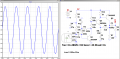#### Attachments

• 2.8 KB Views: 5
Last edited:
•Man789

#### Man789

Joined Jan 26, 2023
10

#### Man789

Joined Jan 26, 2023
10
The main mistake of novice amplifier-builders is not taking into account the impedance of the signal source. In an electret microphone, this impedance is equal to the value of the load resistor. If you add this impedance, you will have an even lower signal at the load. If you amplify the transistor to 200 (at 20mA), the input impedance of the common emitter stage will be ~200*30mV/20mA=300 ohms. If the impedance of the signal source is about 15 kOhm, we get a resistive divider with a gain of 0.02 ! Install LTspice and download my library of models and symbols and you have a complete electronic circuit simulation system.
See in LTspice.View attachment 286201

I tried to simulate the circuit using ngspice... sadly I couldn't install LTSpice as I am using Linux, tomorrow I will install Windows in another computer and try it.

Nonetheless I have a couple of questions, as my simulated output is not as yours. Here my circuit and my output: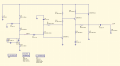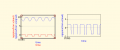As you can see the signal is cut on the top side and the output seems a square wave.

Could you explain my a little about your condenser microphone design? I think my error is on this device.
I have several questions:
1. What is the frequency 1k Hz? Is it voltage, current or sound wave?
2. What does SPL stand for? I guess dB stands for decibel.
3. Sens as I understand stands for sensitivity. Is the units of this constant dB as well?
4. RLoad is a resistance connected to the 2nd terminal of the microphone? To the source of the JFET?
5. If you know what I am missing on my copy of your design it would be nice to know.